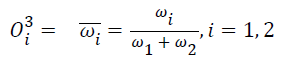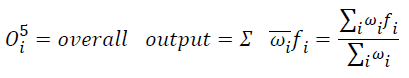## Biomedical Research

All submissions of the EM system will be redirected to Online Manuscript Submission System. Authors are requested to submit articles directly to Online Manuscript Submission System of respective journal.

Research Article - Biomedical Research (2018) Volume 29, Issue 14

## Lung cancer segmentation and diagnosis of lung cancer staging using MEM (modified expectation maximization) algorithm and artificial neural network fuzzy inference system (ANFIS)

Lavanya M* and Muthu Kannan P

Department of Electrical and Electronics Engineering, Saveetha School of Engineering, Saveetha Institute of Medical and Technical Sciences, Saveetha University, Thandalam, Chennai, India

*Corresponding Author:
Lavanya M
Department of Electrical and Electronics Engineering
Saveetha School of Engineering
Saveetha Institute of Medical and Technical Sciences
Saveetha University, Thandalam, Chennai, India

Accepted date: June 29, 2018

Visit for more related articles at Biomedical Research

### Abstract

Medical image processing plays a major role in advancing treatment planning, identification of disease and guiding for surgery. Lung cancer is one among the dangerous diseases that leads to death of most human beings due to uncontrolled growth in the cell. This research area is finding more importance among researchers is that because the available methods for lung cancer detection are very painful. So the automatic detection of lung nodule of computer tomography (CT) is finding more importance among researchers. Though there are a number of methods available for segmentation of lung nodules each method has its drawbacks because of the divergence that exists in lung nodule. The proposed method is the detection of lung nodules using MEM algorithm and classification is based on Artificial Neural Network Fuzzy Inference System (ANFIS) experimental results illustrate in an improvement of accuracy and reduction of false positive results. A comparative analysis is performed with FCM and FLICM algorithms to show the superior nature of MEM.

## Keywords

CT, ANFIS, Nodule, MEM algorithm, Segmentation.

## Introduction

Lung cancer has become one of the leading causes of death rates all over the world [1,2]. Cancer is caused because of uncontrolled cell growth if not treated in time it spreads throughout the body and results in death. The major cause of cancer is due to smoking and secondary cause is continuous exposure to air pollution, radon gas, gene mutation and secondhand smoking . The major symptoms include chest pain due to a continuous cough that extends to weight loss, weakness, shortness of breath [4,5]. There are various advanced techniques that are followed for treating cancer chemotherapy, radiation therapy, and surgery. However, these treatments are very painful [6-9]. So to overcome this biomedical image processing plays a vital role when the diagnostic test is very simple and improves the survival rate of the people.

Though the technique of automatic lung nodule is tough to perform there is always a way for improvement [10,11]. The CT images are collected from the LIDC database and image noise is removed through pre-processing step and the lung cancer nodule is detected and finally, the lung cancer stage is classified [12-16].

Segmentation methods are classified into seven major types in this work clustering method of segmentation is considered [17-19]. Several clustering methods are implemented still some room for improvement is always required. The clustering methods that are already implemented are discussed below.

Deen  proposed Fuzzy C-Means (FCM), a technique applied when clustering is needed, and this technique allows one piece of data to belong to more than one cluster by assigning a membership value of each data pattern to each cluster. Assigned membership values are between 0 to 1 and membership values for each data from all the clusters will add to 1. But the system has the following drawbacks like the prior specification of the number of clusters; the number of iterations is more.

Kumar  proposed K-means clustering technique, it is a clustering method used for segmentation by dividing the objects into k groups first by calculating the mean than computing the distance between each point from the cluster mean. The main drawback of this method is firstly it is difficult to predict the k-value. For different initial partitions, different results are produced, it does not produce good results for different size and different density clusters and finally, it doesn’t work well with global clusters.

Wau  proposed Mean Shift Segmentation Algorithm it works by defining a window around it and then calculating mean of the data point. This uses kernel approach for calculating the density of the gradient point. The drawbacks of this method are the output depends on the window size and the computation is expensive and it does not scale well with a dimension of feature space.

Yiming  proposed the Expectation Maximization algorithm; it is an iterative procedure which is used to compute maximum likelihood estimates for the missing or hidden data. In this method, the model parameters are predicted from the most likely data. Each iteration consists of two steps: E-step that estimated missing data of the model parameters-steplikelihood data is maximized by assuming the missing data are identified. The main drawback is it has slow convergence in some cases.

To overcome the above drawbacks a new method is proposed Modified Expectation Maximization where Comparison of a pixel to a pixel is done using a replicated matrix for the hidden data’s calculation. So the segmentation result is more accurate when compared to other techniques.

## Proposed Method

Figure 1 shows the proposed method obtained images from the database (LDIC) further the images are enhanced using a technique adaptive equalization and noise removal is done using median filter and segmentation of the nodule is carried out using MEM algorithm and feature extraction is done and disease classification is done using ANFIS classifier.

Image enhancement using adaptive histogram equalization

Adaptive histogram equalization has found its importance in application because of its efficiency and simplicity . It enhances the image and improvises the visual effects by enlarging the gray distribution of the image according to its probability distribution function. It overcomes the drawbacks of the existing histogram method by automatically identifying and adapting the gray level of the image. Figure 2 shows the original image. Figure 3 shows the enhanced image through histogram equalization.

Noise removal using median filtering

The median filter is used for reducing noise, particularly impulsive noise and salt and pepper noise . It also reduces random noise and it pertains to the edge information of the image. It replaces the pixel with the median of all pixels of its neighborhoods (ω). Figure 4 shows the pre-processed image.

y (m, n)=median (x (i, j), (i, j) ɛ ω)

Segmentation using MEM (modified expectation maximization) algorithm

MEM algorithm calculated value is related to the segment depend on the weights how the calculated value is completely related to the segregated portion. Comparison of a pixel to a pixel is done using a replicated matrix for the hidden data’s calculation. So the segmentation result is more accurate when compared to other techniques. Figure 5 shows the lung nodule segmented using the MEM segmentation method.

1. MEM algorithm consists of following steps:

2. First initialize expectation and the maximization step parameters.

3. Then increase the maximization step by one.

4. Next step is to concatenate the input image.

5. Replace expectation and maximization matrices.

6. Find out the difference between the replicated and input image.

7. Calculate the new expectation and maximization step position.

8. Iterate the expectation and maximization step value.

Feature extraction

After the completion of the segmentation process, the segmented nodule is utilized for feature extraction process which acts as one of the important steps for providing more detailed information about the image . The physical features extracted are:

1. Area: Area represents the aggregate of the area of a pixel value in the image that is normally registered as 1 in the given binary image A=n (1).

2. Perimeter: The perimeter represents the total count of pixels at the edge of the given object.

3. Eccentricity: It represents the distance between the major axis and the foci of the ellipse.

Classification using ANFIS classifier

ANFIS is a neural network based approach which is a datadriven process . This is based on a clustering approach where training sets are clustered for an unknown function. Figure 6 shows the ANFIS classifier.

The individual layer function is described below:

Layer 1: Every node in this layer ‘i’ is adaptive with the node function

Oi1=μAi (x)

x: Input node.

Ai: The linguistic variable associated with node function.

μA: Membership function of AiμAi (x) is chosen as:

μAi (x)=1/((1+((x-ci)/ai)2) bi)

μAi (x)=exp (-((x-ci)/ai)2)

Where x is the input and (ai,bi,ci) is the premise parameter set.

Layer 2: Each node in layer 2 is a fixed node that calculates the firing strength wi.

The output of nodes in this layer is a product of incoming signals to it, expressed as:

Oi2=wi=μAi (x) μBi (y), i=1, 2

Layer 3: Each node is a fixed node in this layer. Each node calculates ration of ith firing strength of sum of firing strength. The output of this node is expressed as:Layer 4: Each node in this layer is an adaptive node. Node function is expressed as:Layer 5: Only one fixed node is available in this layer, the overall output is a summation of all incoming signals.## Results and Discussion

The image segmentation process is done using a MEM segmentation method using MATLAB version14. For performance analysis the various parameters are discussed .

Sensitivity=True positive/(True positive+False negative)

Specificity=True negative/(True negative+False positive)

Accuracy=(True positive+True negative)/(True positive+False negative+True negative+False positive)

Positive predictive value (Ppv)=True positive/(True positive +False negative)

The performance parameters of the proposed system are tabulated which represents the accuracy of training data sets. Table 1 shows the performance measures of the proposed system when compared with existing systems like FCM and FLICM. T1 indicates stage 1 cancer; T2 indicates stage 2 cancer; T3 indicates stage-3 cancer; T4 indicates stage 4 cancer.

Performance parameters Sensitivity Specificity Precision Accuracy
FCM segmentation and BPN classification 0.9087 0.92 0.927 0.859
FLICM segmentation and SVM classification 0.75 0.84775 0.9 0.9
MEM segmentation and ANFIS classification 0.82 0.69 0.97 0.95

Table 1: Comparison of performance measures of the proposed system with existing system.

Figure 7 (a-c) shows the original image obtained from the LIDC database, the lung nodule segmented image using a MEM segmentation algorithm and the cancer stage result obtained from the training given to ANFIS algorithm based on the data’s obtained through feature extraction of the segmented nodule respectivley.

The sensitivity comparison is shown in Figure 8. The proposed system provides better sensitivity (82%) when compared with SVM (75%) but lesser when compared to BPN (90.8%). The sensitivity of this algorithm will increase with an increase in the number of images.

The specificity comparison of existing with proposing is shown in Figure 9. The existing system BPN (92%) and SVM (84.7%) has less specificity than that of the proposed system ANFIS (69%).

The accuracy comparison is shown in Figure 10. The proposed system ANFIS yields result with better accuracy (95%) when compared with existing BPN (85%) and SVM (90%). With an increase in the number of images accuracy of the proposed system will increase.

The precision comparison of existing method BPN (92%), SVM (90%) and proposed system ANFIS (97%) are shown in Figure 11. The results of the proposed system are good when compared with that of the existing systems.

## Conclusion

In this paper, a unique method for segmentation is tested. The process starts with image enhancement done using histogram equalization followed by pre-processing done by median filtering and segmentation process is done by a MEM segmentation algorithm for further classification for the segmented nodule to detect the severity of the disease is done by ANFIS classifier. This method performs better compared to FCM and FLICM clustering algorithms that is proved by the performance measures of sensitivity 82%, specificity 92%, precision 97% and accuracy 95%. The future work can be extended by hybridization of MEM algorithm with edge detection techniques to further increase the accuracy of segmentation of lung nodule.